# Book Recommendations

 ClojureProgramming Clojureby Stuart Halloway and Aaron Bedra. This is the second edition of the first book on Clojure, and is an excellent introduction.Clojure Programmingby Chas Emerick, Brian Carper, and Christophe Grand. This is excellent introduction into Clojure language, with many examples.Clojure in Actionby Amit Rathore. This book is an introduction into Clojure programming language.The Joy of Clojure: Thinking the Clojure Wayby Michael Fogus and Chris Houser. This book describes more “advanced Clojure programming” and is great companion to any of aforementioned books.Clojure Data Analysis Cookbook– this book contains over 110 recipes to help you dive into the world of practical data analysis using Clojure, including how to use Incanter for this task. You can read sample chapter to get more information about this book. General statisticsThe Lady Tasting Tea: How Statistics Revolutionized Science in the Twentieth CenturyBy David Salsburg. An entertaining introduction to the history of statistics in the twentieth century, and how it influenced science. R booksThe R BookBy Michael Crawley. This BIG book covers more of R’s features than anything else to date. It isn’t perfect, but it is a useful reference.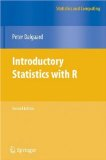Introductory Statistics with R (Statistics and Computing)By Peter Dalgaard. A really nice introduction to R.Software for Data Analysis: Programming with R (Statistics and Computing)By John Chambers. The best book on programming R, by the man behind S (the forerunner of R). Most books approach R as a statistical environment, this one talks about it as a programming language.Data Manipulation with R (Use R)By Phil Spector. Great book on using R to manipulate data.R Graphics (Computer Science and Data Analysis)By Paul Murrell. A great book covering both R’s traditional graphics system and the Lattice graphics system. Matrix algebra for statistics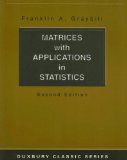Matrices with Applications in Statistics (Duxbury Classic)By Franklin Graybill. A great introduction to the matrix algebra necessary for many statistical techniques. Old, but still great.Matrix Algebra: Theory, Computations, and Applications in Statistics (Springer Texts in Statistics)By James Gentle. Another excellent introduction to the matrix algebra needed for statistics, but with more emphasis on numerical methods than Graybill’s book. Bayesian statistics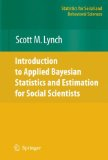Introduction to Applied Bayesian Statistics and Estimation for Social Scientists (Statistics for Social and Behavioral Sciences)By Scott Lynch. This is the best introduction to using Bayesian methods and MCMC ever, and it has great example R code. The R and WinBugs code and data from the book are available at Scott Lynch’s homepage.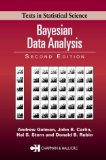Bayesian Data Analysis, Second Edition (Texts in Statistical Science)By Andrew Gelman. A next book after Scott Lynch’s, one of the standard texts. Also check out Andrew Gelman’s fantastic blog, Statistical Modeling, Causal Inference, and Social Science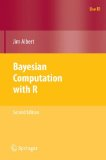Bayesian Computation with RBy Jim Albert. Another good introduction to Bayesian statistics with actual code examples in R. Many of the code examples rely on an R library by the author, which is available for download at the book’s website.Ordinal Data Modeling (Statistics for Social and Behavioral Sciences)By Valen Johnson & James Albert. Another good introduction to Bayesian (and classical) modeling, focusing on ordinal data in the social sciences. The Matlab code and data for the book’s examples are available online at James Albert’s homepage Machine learning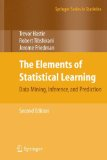The Elements of Statistical Learning: Data Mining, Inference, and Prediction, Second Edition (Springer Series in Statistics)by Hastie, Tibshirani, and Friedman. The classic book on statistical learning techniques by three of the best in the field.Pattern Recognition and Machine Learning (Information Science and Statistics)By Christopher Bishop. A great introduction to the fundamentals of machine learning techniques.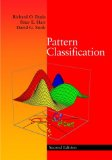Pattern Classification (2nd Edition)By Richard Duda. A great introduction and survey of machine learning techniques. A more applied approach than Bishop’s book with less of the theory behind the techniques. Causal modelingStructural Equations with Latent VariablesBy Ken Bollen. The classic text on structural equation modeling, and very well written.Causality: Models, Reasoning, and InferenceBy Judea Pearl. The best introduction to causal modeling with DAGs (directed acyclic graphs).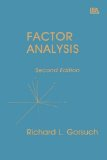FACTOR ANALYSISBy Richard Gorsuch. Old, but my favorite introductory text on factor analysis, and a classic. Data Visualization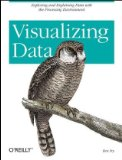Visualizing Data: Exploring and Explaining Data with the Processing Environment By Ben Fry, this is a great introduction to Processing, and one that emphasizes data visualization.Processing: A Programming Handbook for Visual Designers and ArtistsBy Casey Reas and Ben Fry, this is definitive reference for the Processing language.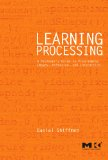Learning Processing: A Beginner’s Guide to Programming Images, Animation, and Interaction (Morgan Kaufmann Series in Computer Graphics)By Daniel Shiffman, this is a fantastic introduction to graphics programming using Processing. Teaching statisticsTeaching Statistics: A Bag of TricksBy Andrew Gelman and Deborah Nolan. A nice set of examples for teaching statistics to students.